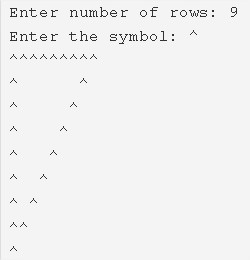# C Program Hollow Inverted Right Triangle Star Pattern

C program to print a hollow inverted right triangle star pattern – In this article, we will discuss the multiple methods to print a hollow inverted right triangle star pattern.

Suitable examples and sample programs have also been added so that you can understand the whole thing very clearly. The compiler has also been added with which you can execute it yourself.

The methods used to do so in this piece are as follows:

• Using For Loop
• Using While Loop
• Using Do-While Loop

As the name of the title suggests, in this piece, a right triangle is a triangle with a single angle being 90 degrees.As you can see, firstly, you need to enter the number of rows. Depending on that, you will be able to print the hollow inverted right triangle with that particular height.

Thus, the number of ways you can print the same in C programming is as follows:

## Using For Loop

1. Read the entered number of rows, character and store those values into the variables n, ch.

2) The outer for loop iterates through rows with the structure for(i=n;i>0;i–).

3) For 1st row, nth row

a) The 1st inner for loop iterates through columns with the structure for(j=1;j<=i;j++) and prints symbol.

4) For other than the 1st row, last row

a) The 2nd inner loop iterates through columns with the structure for(j=1;j<=i;j++)

if j=1 or i it prints symbol, otherwise it prints space.

5) Cursor comes to next line for each iteration of outer for loop.

Output:

### Using While Loop

1. The outer while loop iterates through rows until i>0.

2) For 1st row, nth row

The 1st inner while loop iterates through columns until j<=i condition fails.

prints symbol,j value increased by 1.

3) For other than the 1st row, nth row

The 2nd inner while loop iterates through columns until j<=i condition fails.

if j=1 or i it prints symbol. Otherwise, it prints space.

j value increased by 1.

4) Cursor comes to next line.i value decreased by 1.

Output:

#### Using Do-While Loop

1. The Outer do-while loop iterates through rows.

2) For 1st row, nth row

The 1st inner do-while loop iterates through columns.

Prints the symbol,j value increased by 1.

Checks the condition j<=i. If the condition is true then loop iterates again. Loop iterates until the condition fails.

3) For other than the 1st row, nth row

The 2nd inner do-while loop iterates through columns.

prints symbol if j=1 or n.otherwise prints space.

j value increased by 1.

Checks the condition j<=i.If the condition is true then loop iterates again. Repeats until the condition becomes false.

4) Cursor comes to next line.i value decreased by 1.

5) Checks the condition i>0. If the condition is true then loop iterates again. The loop iterates until the condition becomes false.

Output:
x

## C Program To Print Number Of Days In A Month | Java Tutoring

C program to input the month number and print the number of days in that ...